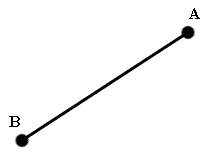Study of mathematics online.
Study math with us and make sure that "Mathematics is easy!"

# Online calculator. Distance between two points calculator

This online calculator will help you to find distance between two points.

Using this online calculator, you will receive a detailed step-by-step solution to your problem, which will help you understand the algorithm how to find midpoint between two points.

## Distance calculatorSelect dimension:

Dimension:

Input points coordinates:

A: (
,,
)
B: (
,,
)

You can input only integer numbers, decimals or fractions in this online calculator (-2.4, 5/7, ...). More in-depth information read at these rules.